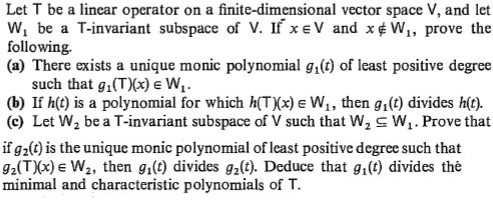# Let T be a linear operator on a finite-dimensional vector space V, and letw, be a T-invariant subspace of V. If xe V and x W,, prove thefollowing.(a) There exists a unique monic polynomial g,(1) of least positive degreesuch that g,(T)(x) e W,.(b) If h(t) is a polynomial for which h(TXx) e W1, then g,(t) divides h(t).(c) Let W, be a T-invariant subspace of V such that W, s W,. Prove thatif g2(t) is the unique monic polynomial of least positive degree such that92(T)(x) e W2, then g,(t) divides g,(f). Deduce that g,(t) divides theminimal and characteristic polynomials of T.

Question
1 viewshelp_outlineImage TranscriptioncloseLet T be a linear operator on a finite-dimensional vector space V, and let w, be a T-invariant subspace of V. If xe V and x W,, prove the following. (a) There exists a unique monic polynomial g,(1) of least positive degree such that g,(T)(x) e W,. (b) If h(t) is a polynomial for which h(TXx) e W1, then g,(t) divides h(t). (c) Let W, be a T-invariant subspace of V such that W, s W,. Prove that if g2(t) is the unique monic polynomial of least positive degree such that 92(T)(x) e W2, then g,(t) divides g,(f). Deduce that g,(t) divides the minimal and characteristic polynomials of T. fullscreen

### This question hasn't been answered yet.